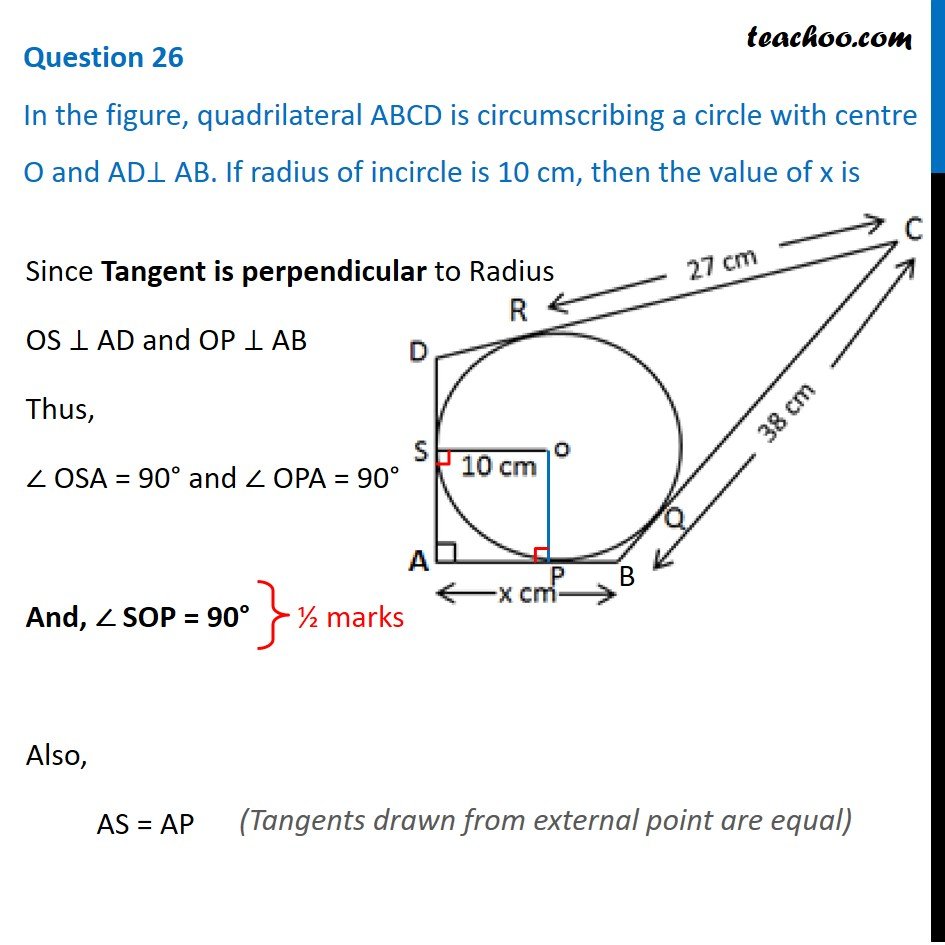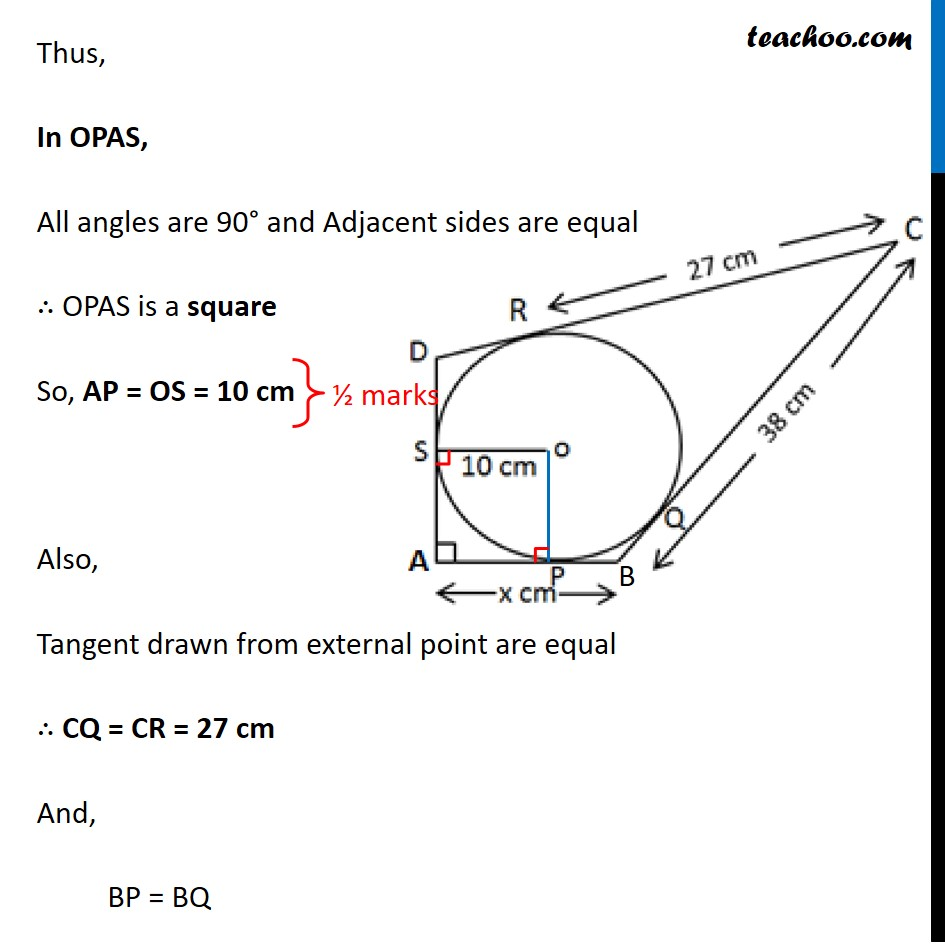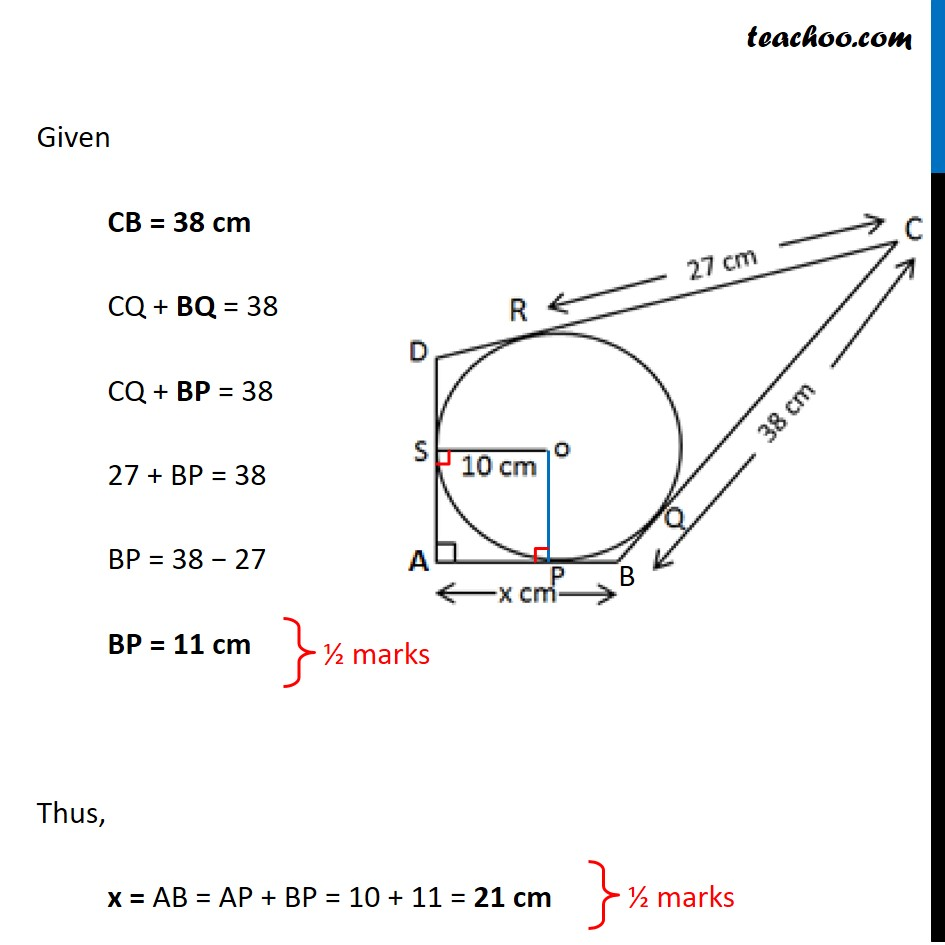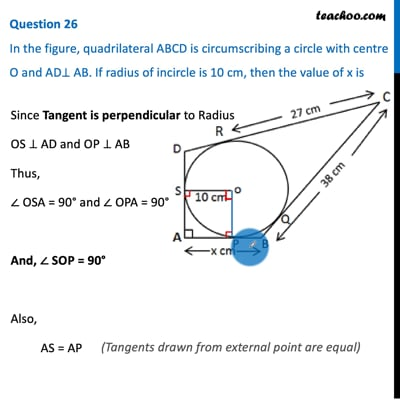CBSE Class 10 Sample Paper for 2021 Boards - Maths Standard

Class 10
Solutions of Sample Papers for Class 10 Boards

## In the figure, quadrilateral ABCD is circumscribing a circle with centre O and AD⊥AB. If radius of incircle is 10cm, then the value of x isThis video is only available for Teachoo black users

Learn in your speed, with individual attention - Teachoo Maths 1-on-1 Class

### Transcript

Question 26 In the figure, quadrilateral ABCD is circumscribing a circle with centre O and AD⊥ AB. If radius of incircle is 10 cm, then the value of x is Since Tangent is perpendicular to Radius OS ⊥ AD and OP ⊥ AB Thus, ∠ OSA = 90° and ∠ OPA = 90° And, ∠ SOP = 90° Also, AS = AP (Tangents drawn from external point are equal) Thus, In OPAS, All angles are 90° and Adjacent sides are equal ∴ OPAS is a square So, AP = OS = 10 cm Also, Tangent drawn from external point are equal ∴ CQ = CR = 27 cm And, BP = BQ Given CB = 38 cm CQ + BQ = 38 CQ + BP = 38 27 + BP = 38 BP = 38 − 27 BP = 11 cm Thus, x = AB = AP + BP = 10 + 11 = 21 cm